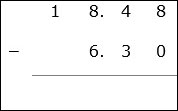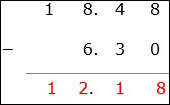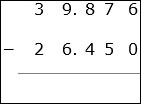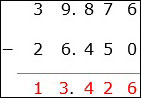# Subtraction of Aligned Decimals

Decimals have to be aligned so that digits with same place values are placed one above the other. Such decimals are aligned decimals. The decimals have to be aligned before subtraction is done. Subtraction of aligned decimals is similar to subtraction of whole numbers.

• First the decimals are aligned so that digits of same place values are placed above and below each other.

• We then add zeros to the right of decimal numbers where ever necessary so that all numbers have the same number of digits after the decimal point.

• We begin with the rightmost column and start subtracting the digits.

• Then we keep moving to the left till all columns are subtracted.

Subtract 18.48 − 6.3

### Solution

Step 1:The decimals are aligned as shown. A zero is added to the right of 6.3 as the maximum number of decimal places is 2.

Step 2:

We start with the right most column. Subtract 8 − 0 = 8; then moving to left column 4 − 3 = 1; again moving left 8 − 6 = 2; next 1 − 0 = 1;

Step 3:

So the difference of the decimals is 12.18Subtract 39.876 − 26.45

### Solution

Step 1:The decimals are aligned as shown. A zero is added to the right of 26.45 as the maximum number of decimal places is 3.

Step 2:

We start with the right most column. Subtract 6 − 0 = 6; then moving to left column 7 − 5 = 2; again 8 − 4 = 4; next 9 − 6 = 3.; 3 – 2 = 1

Step 3:

So the difference of the decimals is 13.426